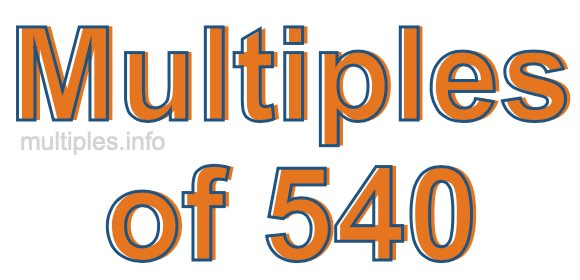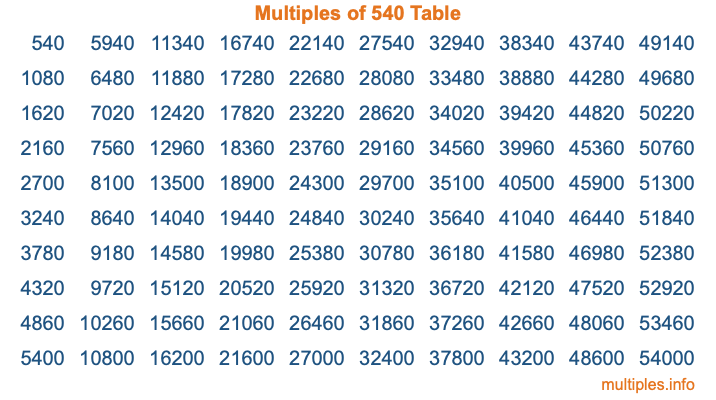Multiples of 540Welcome to the Multiples of 540 page. Here we will first teach you everything you will ever need to know about the multiples of 540, and then give you a study guide summary of everything we taught you to make sure you remember it all. Use this page to look up facts and learn information about the multiples of 540. This page will make you a multiples of five hundred forty expert!

Definition of Multiples of 540
Multiples of 540 are all the numbers that when divided by 540 equal an integer. Each of the multiples of 540 are called a multiple. A multiple of 540 is created by multiplying 540 by an integer.

Therefore, to create a list of multiples of 540, you start with 1 multiplied by 540, then 2 multiplied by 540, then 3 multiplied by 540, and so on for as long as you want. Thus, the list of the first five multiples of 540 is 540, 1080, 1620, 2160, and 2700. To see a larger list of multiples of 540, see the printable image of Multiples of 540 further down on this page. We also have a category where you can choose any nth multiple of 540.

Multiples of 540 Checker
The Multiples of 540 Checker below checks to see if any number of your choice is a multiple of 540. In other words, it checks to see if there is any number (integer) that when multiplied by 540 will equal your number. To do that, we divide your number by 540. If the the quotient is an integer, then your number is a multiple of 540.

Is  a multiple of 540?

Least Common Multiple of 540 and ...
A Least Common Multiple (LCM) is the lowest multiple that two or more numbers have in common. This is also called the smallest common multiple or lowest common multiple and is useful to know when you are adding our subtracting fractions. Enter one or more numbers below (540 is already entered) to find the LCM.

Check out our LCM Calculator if you need more details about the Least Common Multiple or if you need the LCM for different numbers for adding and subtraction fractions.

nth Multiple of 540
As we stated above, 540 is the first multiple of 540, 1080 is the second multiple of 540, 1620 is the third multiple of 540, and so on. Enter a number below to find the nth multiple of 540.

th multiple of 540

Multiples of 540 vs Factors of 540
540 is a multiple of 540 and a factor of 540, but that is where the similarities end. All postive multiples of 540 are 540 or greater than 540. All positive factors of 540 are 540 or less than 540.

Below is the beginning list of multiples of 540 and the factors of 540 so you can compare:

Multiples of 540: 540, 1080, 1620, 2160, 2700, etc.

Factors of 540: 1, 2, 3, 4, 5, 6, 9, 10, 12, 15, 18, 20, 27, 30, 36, 45, 54, 60, 90, 108, 135, 180, 270, 540

As you can see, the multiples of 540 are all the numbers that you can divide by 540 to get a whole number. The factors of 540, on the other hand, are all the whole numbers that you can multiply by another whole number to get 540.

It's also interesting to note that if a number (x) is a factor of 540, then 540 will also be a multiple of that number (x).

Multiples of 540 vs Divisors of 540
The divisors of 540 are all the integers that 540 can be divided by evenly. Below is a list of the divisors of 540.

Divisors of 540: 1, 2, 3, 4, 5, 6, 9, 10, 12, 15, 18, 20, 27, 30, 36, 45, 54, 60, 90, 108, 135, 180, 270, 540

The interesting thing to note here is that if you take any multiple of 540 and divide it by a divisor of 540, you will see that the quotient is an integer.

Multiples of 540 Table
Below is an image of the first 100 multiples of 540 in a table. The table is in chronological order, column by column. The first column has the first ten multiples of 540, the second column has the next ten multiples of 540, and so on.The Multiples of 540 Table is also referred to as the 540 Times Table or Times Table of 540. You are welcome to print out our table for your studies.

Negative Multiples of 540
Although not often discussed or needed in math, it is worth mentioning that you can make a list of negative multiples of 540 by multiplying 540 by -1, then by -2, then by -3, and so on, to get the following list of negative multiples of 540:

-540, -1080, -1620, -2160, -2700, etc.

Multiples of 540 Summary
Below is a summary of important Multiples of 540 facts that we have discussed on this page. To retain the knowledge on this page, we recommend that you read through the summary and explain to yourself or a study partner why they hold true.

There are an infinite number of multiples of 540.

A multiple of 540 divided by 540 will equal a whole number.

540 divided by a factor of 540 equals a divisor of 540.

The nth multiple of 540 is n times 540.

The largest factor of 540 is equal to the first positive multiple of 540.

540 is a multiple of every factor of 540.

540 is a multiple of 540.

A multiple of 540 divided by a divisor of 540 equals an integer.

540 divided by a divisor of 540 equals a factor of 540.

Any integer times 540 will equal a multiple of 540.

Multiples of a Number
Here you can get the multiples of another number, all with the same attention to detail as we did for multiples of 540 on this page.

Multiples of
Multiples of 541
Did you find our page about multiples of five hundred forty educational? Do you want more knowledge? Check out the multiples of the next number on our list!